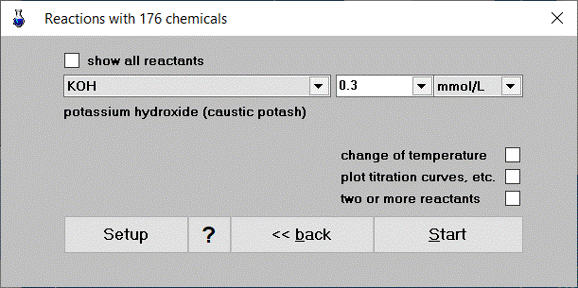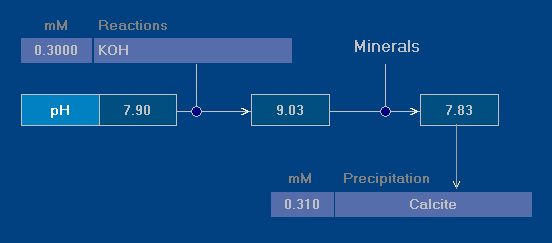# Addition of Potassium Hydroxide (KOH)

Problem

Given is a water with pH 7.9 (example water calcite-3.sol).

• What is the pH after addition of 0.3 mmol/L KOH?
• What is the impact of calcite precipitation?Click Open and select the example water calcite-3.sol. Click Reac and enter for “KOH” the value 0.3 mmol/L.

Run the calculation with Start. The result is:It shows how the pH changes from 7.9 (initial solution) to 9.03 or 7.83, depending on whether (precipitation of) mineral phases is considered or not:

 pH = 9.03 (without calcite precipitation) pH = 7.83 (with calcite precipitation)

That is, two equilibrium calculations were performed. The first one ignores mineral phases at all (output 1); the second one considers phase equilibria and allows precipitation when one or more minerals are supersaturated (output 2).

In this example the solution is supersaturated with calcite (CaCO3), thus calcite precipitates (and alters the pH value).

Amount of Precipitated Calcite

A closer look at the output table (next window that opens after the above screenshot) reveals that the solutions differ in four parameters (concentration units in mM):

 parameter input water output 1 output 2 (no precipitation) (with CaCO3 precipitation) pH 7.90 9.03 7.83 Ca 1.22 1.22 0.91 DIC 2.46 2.46 2.15 K 0.05 0.35 0.35

In fact, the difference of the Ca value in the last two columns gives the molar amount of precipitated calcite:

 (1) CaCO3  =  1.22 mM – 0.91 mM  =  0.31 mM

Exactly the same result is obtained from the DIC values:

 (2) CaCO3  =  2.46 mM – 2.15 mM  =  0.31 mM

Calcium Carbonate Saturation State

Each water is characterized by a set of parameters controlling the CaCO3 saturation state. In the present case we would find the following information:1

 SI_Calcite = 1.29   (saturation index) Calcium Carbonate Precipitation Potential (CCPP): 0.30 mmol/L 29.7 mg/L calcite precipitates.

The value of CCPP tells us how much calcite precipitates. But this value (0.30 mM does not exactly match the amount predicted in Eq.(1) or Eq.(2). What’s going on?

The answer is simple. The parameters of the CaCO3 saturation state are determined for the evaluation temperature Teval = 5.5 which differs from the temperature T = 20 of the water sample (both temperatures can be set in the input window separately). What we have is:

 (3a) amount of precipitated calcite at T = 20: 0.31 mM (3b) amount of precipitated calcite at T = 5.5: 0.30 mM

It’s easy to check this statement. Enter Teval = 20 in the input panel and rerun the calculation. You will get CCPP = 0.31 mM.

Footnotes

1. Click next » in the bottom menu of the “Output table” window.